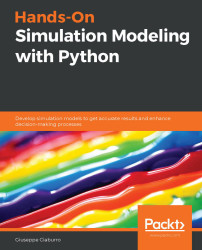•#### Hands-On Simulation Modeling with Python#### Overview of this book

Simulation modeling helps you to create digital prototypes of physical models to analyze how they work and predict their performance in the real world. With this comprehensive guide, you'll understand various computational statistical simulations using Python. Starting with the fundamentals of simulation modeling, you'll understand concepts such as randomness and explore data generating processes, resampling methods, and bootstrapping techniques. You'll then cover key algorithms such as Monte Carlo simulations and Markov decision processes, which are used to develop numerical simulation models, and discover how they can be used to solve real-world problems. As you advance, you'll develop simulation models to help you get accurate results and enhance decision-making processes. Using optimization techniques, you'll learn to modify the performance of a model to improve results and make optimal use of resources. The book will guide you in creating a digital prototype using practical use cases for financial engineering, prototyping project management to improve planning, and simulating physical phenomena using neural networks. By the end of this book, you'll have learned how to construct and deploy simulation models of your own to overcome real-world challenges.
PrefaceSection 1: Getting Started with Numerical SimulationFree Chapter
Chapter 1: Introducing Simulation ModelsChapter 2: Understanding Randomness and Random NumbersChapter 3: Probability and Data Generation ProcessesSection 2: Simulation Modeling Algorithms and TechniquesChapter 4: Exploring Monte Carlo SimulationsChapter 5: Simulation-Based Markov Decision ProcessesChapter 6: Resampling MethodsChapter 7: Using Simulation to Improve and Optimize SystemsSection 3: Real-World ApplicationsChapter 8: Using Simulation Models for Financial EngineeringChapter 9: Simulating Physical Phenomena Using Neural NetworksChapter 10: Modeling and Simulation for Project ManagementChapter 11: What's Next?Other Books You May Enjoy# The pseudorandom number generator

The generation of real random sequences using deterministic algorithms is impossible: at most, pseudorandom sequences can be generated. These are, apparently, random sequences that are actually perfectly predictable and can be repeated after a certain number of extractions. A PRNG is an algorithm designed to output a sequence of values that appear to be generated randomly.

## The pros and cons of a random number generator

A random number generation routine must be the following:

• Replicable
• Fast
• Not have large gaps between two generated numbers
• Have a sufficiently long running period
• Generate numbers with statistical properties that are as close as possible to ideal ones

The most common cons of random number generators are as follows:

• Numbers not uniformly distributed
• Discretization of the generated numbers
• Incorrect mean or variance
• Presence of cyclical variations